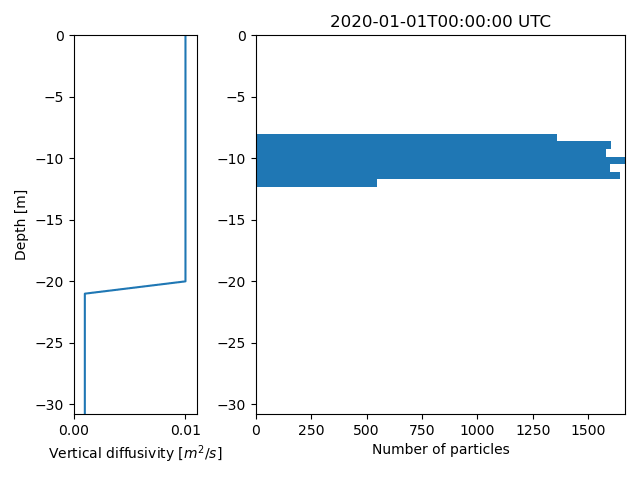# Vertical mixing

```import numpy as np
from datetime import datetime, timedelta
from opendrift.models.oceandrift import OceanDrift
```

Configuration. Edit this section to see the differences.

```N = 10000  # Number of particles
seed_depth = -10 # meters
hours = 2  # Number of hours to mix particles
sea_floor_depth = 100  # m
timestep_seconds = 60  # Timestep for vertical mixing

terminal_velocity = 0  # Neutral particles
#terminal_velocity = 0.005  # Rising particles
#terminal_velocity = -0.005  # Sinking particles

# Profile of diffusivities
z = np.arange(0, -40, -1)

diffusivity = np.ones(z.shape)*.01  # Constant diffusivity
diffusivity[z<-20] = 0.001  # uncomment to reduce mixing below 20m
```

Preparing mixing timestep

```time = datetime(2020, 1, 1, 0)
o = OceanDrift(loglevel=20)
o.set_config('drift:vertical_mixing', True)
o.set_config('vertical_mixing:diffusivitymodel', 'environment')
o.set_config('vertical_mixing:timestep', timestep_seconds)
o.seed_elements(lon=4, lat=60, z=seed_depth, time=time, number=N, terminal_velocity=terminal_velocity)
o.time = time
o.time_step = timedelta(hours=hours)
o.release_elements()
o.environment = np.array(np.ones(N)*sea_floor_depth,
dtype=[('sea_floor_depth_below_sea_level', np.float32)]).view(np.recarray)
o.environment_profiles = {
'z': z,
'ocean_vertical_diffusivity':
np.tile(diffusivity, (N, 1)).T}
```
```13:59:41 INFO    opendrift.models.basemodel:539: OpenDriftSimulation initialised (version 1.10.7 / v1.10.6-119-g1da5bec)
```

Calculate vertical mixing, and return particle depths at all positions

```print('Calculating...')
depths = o.vertical_mixing(store_depths=True)

print('Making animation...')
o.animate_vertical_distribution(depths=depths)
```
```Calculating...
Making animation...
13:59:42 INFO    opendrift.models.basemodel:5350: Saving animation to /root/project/docs/source/gallery/animations/example_vertical_mixing_0.gif...
13:59:48 INFO    opendrift.models.oceandrift:686: Time to make animation: 0:00:06.595846
```Total running time of the script: (0 minutes 7.238 seconds)

Gallery generated by Sphinx-Gallery